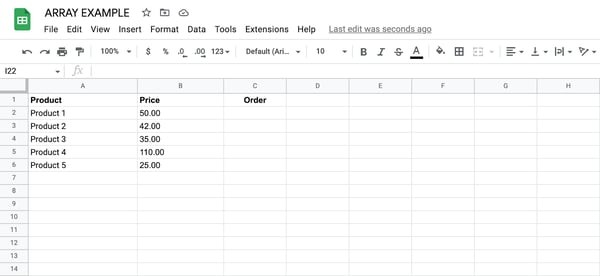# How to Use Arrays in Google Sheets

Google Sheets is a powerful tool for managing and analyzing data, but as your spreadsheets become more complex, you may need more advanced tools to perform calculations and analysis.

Arrays are one such feature. This function in Google Sheets allows you to perform complex calculations across multiple cells or ranges of cells.

Using arrays in Google Sheets can be daunting for beginners, but with a little practice, they can be a game-changer for managing and analyzing data. In this blog post, we'll take a deep dive into how to use arrays in Google Sheets, from basic formulas to more advanced techniques.

## What do arrays do in Google Sheets?

Arrays in Google Sheets are used to perform calculations on multiple cells or ranges of cells simultaneously, without having to create a separate formula for each cell.

An array formula is a special type of formula that can be used to perform calculations across multiple cells in a column, row, or even across multiple columns and rows.

Array formulas are surrounded by curly braces { } and are created by typing the formula and then pressing Ctrl + Shift + Enter (on Windows) or Command + Shift + Enter (on Mac).

An array formula is identified by the presence of curly braces { }. The basic syntax of an array formula in Google Sheets is:

{formula(range)}

"Formula" is any valid formula, and "range" is the range of cells to which the formula should be applied.

For example, if you wanted to calculate the sum of the values in cells A1 to A10, you could use the following array formula:

=SUM(A1:A10)

When you enter this formula as an array formula (by pressing Ctrl + Shift + Enter), Google Sheets will automatically apply the formula to all of the cells in the selected range, producing a single result in each cell.

Arrays can also be used with other functions like AVERAGE, MAX, MIN, and COUNT, as well as with logical functions like IF and AND. Using arrays can be a powerful way to perform complex calculations and analyses in Google Sheets.

## The Benefits of Using Arrays in Google Sheets

Like many formulas used in Google Sheets, the main benefit of arrays is the ability to work with data faster and more simply. A few of the specific benefits of arrays include:

• Efficiency. Arrays can perform calculations on multiple cells or ranges of cells simultaneously, saving time and reducing the amount of manual work required.
• Flexibility. Arrays can be used with a wide range of functions and formulas, making it possible to perform complex calculations and analyses in Google Sheets.
• Accuracy. Using arrays can reduce the risk of errors and mistakes that can occur when performing calculations manually or using individual formulas for each cell.
• Simplicity. Arrays can simplify complex formulas by allowing you to perform multiple calculations with a single formula.
• Dynamic range referencing. Arrays can reference a dynamic range of cells, which is useful when working with data that changes or grows over time.
• Improved readability. Arrays can make spreadsheets easier to read and understand by reducing the number of formulas required and by grouping related calculations together.

## How to Use Arrays in Google Sheets

Applying an array to your formula is very straightforward but not very intuitive. Follow these steps to understand how to use an array formula in Google Sheets:

1. Select the range of cells that you want to perform a calculation on. Make sure that the cells are contiguous (i.e., they're all next to each other).
2. Type the formula that you want to use to perform the calculation. For example, if you want to sum the values in the selected cells, you would type "=SUM(" (without the quotes).
3. With the cursor still inside the formula bar, press and hold the Ctrl and Shift keys (on Windows) or the Command and Shift keys (on Mac) on your keyboard.
4. While holding down those keys, press the Enter key. The formula should now be surrounded by curly braces { }.
5. Release the Ctrl + Shift (or Command + Shift) keys.
6. Press Enter to complete the formula.
7. The formula will now be applied to all cells in the selected range, producing a single result in each cell.
8. If you need to edit the array formula, simply click on the cell containing the formula, then click on the formula bar to edit the formula.

Let's say you have a spreadsheet with a list of products and their prices, and you want to calculate the total cost of purchasing a certain number of each product.

You could use an array formula to calculate the total cost for each product and the overall cost.

Here's how that would work.

1. Start by creating a table with the list of products and their prices.
2. In cell C1, enter the number of units you want to purchase for each product. For example, if you want to purchase three units of Product 1, two units of Product 2, and four units of Product 3, you would enter "3" in cell C2, "2" in cell C3, and "4" in cell C4.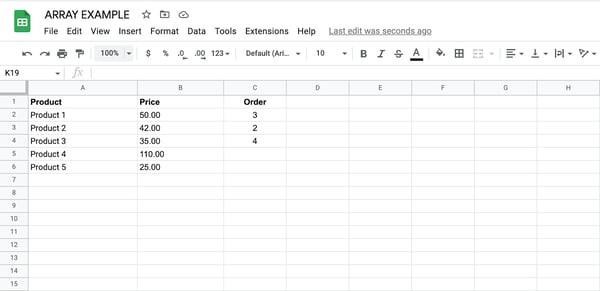3. In cell D1, enter the following formula:

=ArrayFormula(B2:B6*C2:C6)

This formula multiplies the price of each product by the number of units you want to purchase and returns an array of the total cost for each product.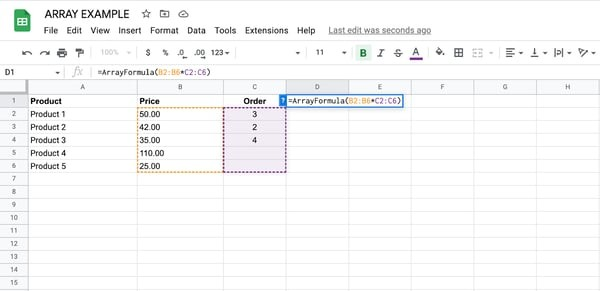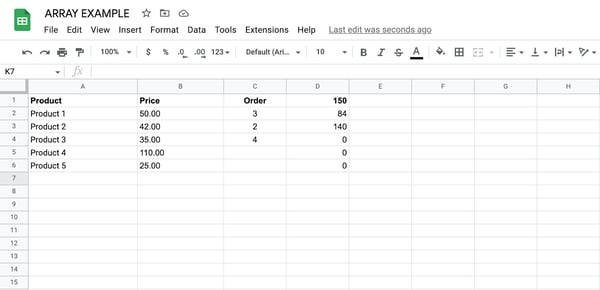4. Finally, in cell D7, enter the following formula:

=SUM(D2:D6)

This formula sums the values in the D2:D6 range to get the total cost of purchasing all the products.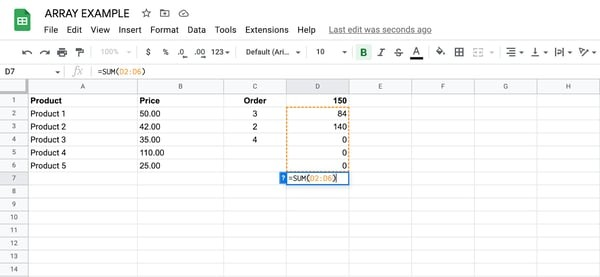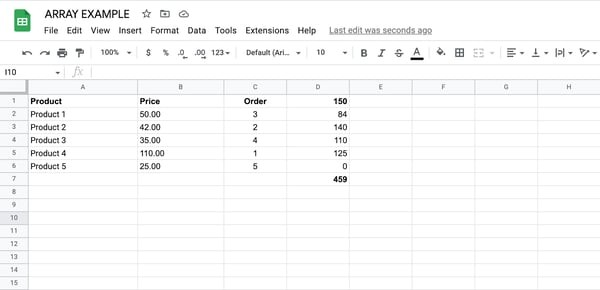5. That's it! Now, as you update the values in column C, the array formula in column D will automatically recalculate the total cost for each product, and the SUM formula in cell D6 will update to reflect the overall cost. This can be a very useful tool for managing and analyzing data in Google Sheets.

## Best Practices for Using Arrays

### Use arrays only when necessary.

While arrays can be a powerful tool for performing complex calculations and analysis in Google Sheets, they can also be resource-heavy and slow down your spreadsheet. Use arrays only when necessary, and try to limit the number of cells they're applied to whenever possible.

### Be careful when editing arrays.

When editing an array formula, be sure to press Ctrl + Shift + Enter (on Windows) or Command + Shift + Enter (on Mac) to apply the changes. If you don't use this key combination, the formula may not work correctly.

### Use named ranges.

Instead of using cell references in your array formulas, consider using named ranges. This can make your formulas easier to read and understand.

Before using an array formula in your spreadsheet, test it on a small set of data to make sure that it's working correctly. This can help you avoid errors and mistakes that can occur when working with large amounts of data.

### Use the IFERROR function.

If your array formula is likely to return an error, consider using the IFERROR function to display a more user-friendly message instead. For example, you could use "=IFERROR(array formula, "No data available")" to display the message "No data available" if the formula returns an error.